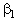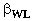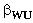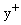# Test Case

## Brief description of the study test case

The study test case concerns the measurements in a controlled diffusion compressor cascade. The main features of the cascade are included in Table 1. The blade coordinates, taken from Elazar and Shreeve (1990) are blade.dat. The coordinate system used is illustrated in Figure 3.

The flow is subsonic (inlet Mach number is about 0.25) and the Reynolds number of the flow is equal to 700,000. Measurements were carried out for three inlet air angles, from design to near stall conditions.

The measurements were conducted at the subsonic cascade wind tunnel of the Naval Postgraduate School. The geometry of the test section is illustrated in Figure 2. Since the blade has been tested in cascade, apart from inlet, exit and stationary walls, all other boundaries are periodic.

The test cascade consists of 20 blades that were cantilevered from a mounting plate attached to the fixed rear side-wall. The inlet air angle () was adjusted by means of the lower end-wall () and the upper end-wall () adjustment, as well as the use of inlet guide vanes (see Figure 2). The test cascade moved horizontally as the end-walls were adjusted.

A four-beam two-colour TS1 model 9100-7 LDV system with oil droplets as seeding was used for velocity measurements in the passage. The measurements were performed in passage formed by two consecutive black–anodised blades. The resulting surface texture was better than 1.5micron. The measuring stations are illustrated in Figure 3.

Verification of the air inlet angle was accomplished by means of a United Sensor Model DA-125 probe at 1.8 chords upstream.

The velocity measurements were verified by hot-wire measurements at several measuring stations.

Forty pressure taps were used to obtain pressure measurements on the blade surfaces.

## Test Case Experiments

Inlet velocity was set to about 85m/s (yielding a Mach number of 0.25). The measured inlet turbulence intensity (as a result of the inlet guide vanes) was 1.4±0.2% in all cases.

The Reynolds number was 700,000 in all cases. The stagnation quantities were 294°K and 1.03atm. The exit static pressure was 1.0atm.

Measurements were carried out for inlet air angles values of 40° (design condition), 43.4° (about twice minimum losses condition) and 46v (near stall condition).

The calculated maximum value of AVDR was 1.05.

Measurements of the normal and the parallel to the blade surface velocity components, as well as of the turbulence intensity were recorded.

Measurements of pressure on the blade surfaces were recorded.

Boundary layer quantities have been calculated by post-processing the measurements.

The quality of the experiments is high, as reported by Elazar and Shreeve (1990):

• The uncertainty in measurement displacement from the blade surfaces is less than 0.05mm.
• The uncertainty in pressure measurements is of the order of 12Pa.
• The uncertainty in velocity measurements is of the order of 0.33% at 10m/s and 0.65% at 100m/s.
• The discrepancy between the probe and LDV measurements at inlet was less than 0.1° for the angle.
• The uniformity discrepancy at inlet is less ±1% for the velocity and 0.75° for the angle.
• The periodicity of the flow downstream of the blades was confirmed.

## TEST CASE EXP–1

This test case corresponds to the flow to the text cascade for an inlet flow angle angle of 40.0o (design conditions). Measurements of the static pressure coefficient along the blade.

exp1.dat (ASCII file; headers: inlet flow angle: 40°, columns: chord-wise position, static pressure coefficient).

## TEST CASE EXP–2

This test case corresponds to the flow to the text cascade for an inlet flow angle angle of 43.4°. Measurements of the static pressure coefficient along the blade.

exp2.dat (ASCII file; headers: inlet flow angle: 43.4°, columns: chord-wise position, static pressure coefficient).

## TEST CASE EXP–3

This test case corresponds to the flow to the text cascade for an inlet flow angle angle of 46.0°. Measurements of the static pressure coefficient along the blade.

exp3.dat (ASCII file; headers: inlet flow angle: 46°, columns: chord-wise position, static pressure coefficient).

## CFD Methods

An overview of the methods used for computations in the study test case follows. Since the flow is low-speed, most of the methods used are elliptic. They are listed below in chronological order.

• The fully elliptic solver of Kang et al. (1995) that is based on the SIMPLE algorithm. A single H-type grid comprising of 158x78 nodes in the stream-wise and span-wise direction respectively was used. Turbulence was modelled by either the low-Reynolds-number k-ε model (Launder and Sharma, 1974) or the Production Term Modification Model (PTMM, Schmidt and Patankar, 1991) that is based on the same model. The value of the first nodes off the solid boundarieswas 0.9~1.2.
• The multi-block algorithm based on collocated grid arrangements of the pressure correction algorithm (Lien et al, 1996). An H-type grid, along with its four-block arrangement, and a four-block arrangement of H-O-type grids were alternatively used (see Figure 4 for their layouts). Two linear low-Reynolds-number k–ε turbulence models (Lauder and Sharma, 1974 and Lien and Leschziner, 1993) and the non-linear variant of Craft et al. (1993) were employed in the computations.
• The low-Reynolds-number k–ω model (Wilcox, 1994) enhanced with Menter’s (1992) modification in the turbulence production term were incorporated in the commercial FLUENT code and the third order accuracy QUICK scheme (Leonard, 1979) for the discretization of the convection terms was employed for computations (Tselepidakis, 1996). A single H-type grid consisting of 234x105 nodes was generated. 132 nodes discretized the blade surfaces. The value of the first nodes off the solid boundarieswas 0.1~2.

Contributors: E. S. Politis - NTUA